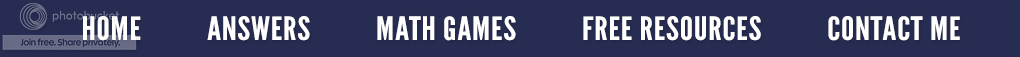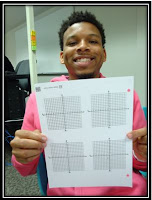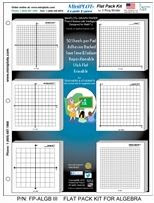### Tools for Helping Students Graph Equations

I work in the math lab at the community college where I also teach. The math lab is staffed by only math instructors and offers free math tutoring to any of our students. We try to have many resources available for our students. When it comes to graphing, we have found that the computer can be very unfriendly. The graphs are often hard to see, and so finding points is next to impossible. We keep in stock some items that help our students.First, we have graph paper that is always available. We keep an assortment of different kinds for our students:
1. 1/4" grid paper
2. Four co-ordinate graphs per page
3. Full co-ordinate graph paper
4. Six small co-ordinate graphs per page
On the right, you see Markwan holding the example of #2.

We also have two-sided white boards. One side is blank while the opposite side contains a coordinate graph outline. Our students make good use of these. They like the fact that they can do the linear or quadratic equation on one side and then construct the graph on the other.  (They don't have to draw the X-Y axes and tick marks for each problem or get out the ruler for accuracy.) Since the white boards are erasable, they can be used over and over again. On the left, Sam is "modeling" the white board. (Both young men wanted to be on my blog and were anxious for me to use their first names.)Click Here for More Information
BUT my favorite item we have on hand are graphing sticky notes.  I often use them in my math classes because students can take notes while drawing examples of graphs and then stick the example right into their math book.  These post-it-notes are called MiniPLOTs®.  They are a unique brand of Post-It Notes designed for math students, teachers and tutors. MiniPLOTs® are 3x3" paper pads with 50 coordinate grid, polar coordinates, or 3D solid shapes printed on each sheet. They are the perfect size for homework and tests. In addition, the company makes them for algebra, geometry, trigonometry, statistics and K-6 math (provides an innovative method of teaching students the basic multiplication and division factors in about six weeks).

These work great when I am grading math homework. When a student has graphed an equation wrong, I simply take a graphing sticky note, correctly graph the equation and stick it beside their incorrect answer. It's important that students see the correct answer so that the wrong one doesn't remain stuck in their heads!

The Math Lab also supplies a reference sheet entitled Graphs of Some Common Functions. It gives an example of the equation being graphed (i.e. f(x) = x ), a visual of the what the graph should look like, the domain, range, and symmetry origin. The students are free to use the laminated ones in the Math Lab, but can also take home a paper one to place in their math notebooks.

On the reverse side of this reference sheet are examples of: Graph of f(x) = ax and Graph of f(x) = loga(x). Besides a visual of the graph, it includes domain, range, decreasing on and horizontal or vertical asymptote.

Most students are visual learners and can see lines and curves and project how they behave intuitively. Their brains can easily understand, understand and recognize pictorially better than just remembering abstract equations.  It is therefore important for students to construct and draw graphs so they can picture them in their minds. Hopefully some of these graphing tools will make constructing those graphs easier.